Courses

# Alphanumeric Series MCQ - 1

## 30 Questions MCQ Test Reasoning Aptitude for Competitive Examinations | Alphanumeric Series MCQ - 1

Description
This mock test of Alphanumeric Series MCQ - 1 for LR helps you for every LR entrance exam. This contains 30 Multiple Choice Questions for LR Alphanumeric Series MCQ - 1 (mcq) to study with solutions a complete question bank. The solved questions answers in this Alphanumeric Series MCQ - 1 quiz give you a good mix of easy questions and tough questions. LR students definitely take this Alphanumeric Series MCQ - 1 exercise for a better result in the exam. You can find other Alphanumeric Series MCQ - 1 extra questions, long questions & short questions for LR on EduRev as well by searching above.
QUESTION: 1

### Directions: Study the following digit-letter-symbol sequence carefully and answer the questions given below: R * T J L 2 \$ D = M # 8 C % B < K 1 & A W ? P E + Q @ 7 F 6  Which of the following is sixth to the left of eighteenth element from the left?

Solution:

18th element from the left of given sequence is 1

And the 6th element to the left of 18th element is 8

QUESTION: 2

### Directions: Study the following digit-letter-symbol sequence carefully and answer the questions given below: R * T J L 2 \$ D = M # 8 C % B < K 1 & A W ? P E + Q @ 7 F 6 How many such numbers are there in the above sequence, each of which is immediately preceded by a consonant and immediately followed by a symbol?

Solution:

R * T J L 2 \$ D = M # 8 C % B < K 1 & A W ? P E + Q @ 7 F 6

The higlighted in bold are two required pairs

QUESTION: 3

### Complete the series: QEU, V9J, 1UF, &JW, ?

Solution:
QUESTION: 4

Directions: Study the following digit-letter-symbol sequence carefully and answer the questions given below:

R * T J L 2 \$ D = M # 8 C % B < K 1 & A W ? P E + Q @ 7 F 6

If the above sequence is written in reverse order then which of the following will be sixth to the right of sixteenth element from the right end?

Solution:
QUESTION: 5

Directions: Study the following digit-letter-symbol sequence carefully and answer the questions given below:

R * T J L 2 \$ D = M # 8 C % B < K 1 & A W ? P E + Q @ 7 F 6

If the first fifteen elements in the above sequence are written in reverse order, then which of the following wil be twenty-first from the right end?

Solution:
QUESTION: 6

Directions (6-10): Study the following arrangement and answer questions given:

648 483 295 333 261 312

Q. If the positions of first and second digits are changed in each number, which is the lowest number?

Solution:

The one having lowest number if 2nd digit.

QUESTION: 7

Study the following arrangement and answer questions given:

648 483 295 333 261 312

Q. If in each number, 1 is added to first digit and then first and third are interchanged, then which is the largest number?

Solution:

648 483 295 333 261 312

adding 1 to each number's first digit gives

748 583 395 433 361 412

swapping first and third digits of eah number

847 385 593 334 163 214

largest number is 847 i.e 648

QUESTION: 8

Study the following arrangement and answer questions given:

648 483 295 333 261 312

Q. In all numbers are arranged in ascending order, then what is the difference between the numbers which is third from the left and second from the right?

Solution:

Ascending Order: 261 295 312 333 483 648
So 483 – 312 = 171

QUESTION: 9

Study the following arrangement and answer questions given:

648 483 295 333 261 312

Q. If one is subtracted from all even digits in the numbers, then in how many numbers, a digit appear twice within a number?

Solution:

Numbers become: 537 373 195 333 151 211
So: 373, 333, 151, and 211.

QUESTION: 10

Study the following arrangement and answer questions given:

648 483 295 333 261 312

Q. What is the resultant if first digit of second highest number is divided by the second digit of the second lowest number?

Solution:

Second highest number = 483
Second lowest number = 295
So resultant = 4/9 = 0.44

QUESTION: 11

Directions (11-15): Study the following arrangement and answer questions given:

B 8 C % S & E K 4 \$ 9 2 ! V A 6 @ T I 8 Z # U 5

Q. Which of the following is fifth to the right of eleventh from the right end of the given series?

Solution:
QUESTION: 12

Study the following arrangement and answer questions given:

B 8 C % S & E K 4 \$ 9 2 ! V A 6 @ T I 8 Z # U 5

Q. If all the symbols are dropped from the series, which number/letter will be 5th to the left of T?

Solution:
QUESTION: 13

Study the following arrangement and answer questions given:

B 8 C % S & E K 4 \$ 9 2 ! V A 6 @ T I 8 Z # U 5

Q. How many symbols are in between 9 and U?

Solution:

!, @, #

QUESTION: 14

Study the following arrangement and answer questions given:

B 8 C % S & E K 4 \$ 9 2 ! V A 6 @ T I 8 Z # U 5

Q. How many numbers are immediately preceeded by a consonant and immediately followed by a symbol?

Solution:

B 8 C % S & E K 4 \$ 9 2 ! V A 6 @ T I 8 Z # U 5

In the given series, K 4 \$ is the only required pair

QUESTION: 15

Study the following arrangement and answer questions given:

B 8 C % S & E K 4 \$ 9 2 ! V A 6 @ T I 8 Z # U 5

Q. Four of the five below follows a certain pattern. Find which one does not follow the pattern.

Solution:

In each pair first two elements are consecutive elements in series and third element is 2 places back the first element in series.
In 8ZT: first two elements are consecutive elements in series and third element is 1 place back the first element.

QUESTION: 16

Directions (16-20): Study the following arrangement and answer questions given:

C ? T F L 6 \$ D % M # 8 R = B ! K 1 & E W * P A + Q @ 7 J 2

Q. How many such numbers are there in the above sequence, each of which is immediately preceded by a consonant and immediately followed by a symbol?

Solution:

L6\$, K1&

QUESTION: 17

Study the following arrangement and answer questions given:

C ? T F L 6 \$ D % M # 8 R = B ! K 1 & E W * P A + Q @ 7 J 2

Q. Which of the following is sixth to the left of eighteenth element from the left?

Solution:
QUESTION: 18

Study the following arrangement and answer questions given:

C ? T F L 6 \$ D % M # 8 R = B ! K 1 & E W * P A + Q @ 7 J 2

Q. If the above sequence is written in reverse order then which of the following will be sixth to the right of sixteenth element from the right end?

Solution:
QUESTION: 19

Study the following arrangement and answer questions given:

C ? T F L 6 \$ D % M # 8 R = B ! K 1 & E W * P A + Q @ 7 J 2

Q. If the first fifteen elements in the above sequence are written in reverse order, then which of the following wil be twenty-first from the right end?

Solution:
QUESTION: 20

Study the following arrangement and answer questions given:

C ? T F L 6 \$ D % M # 8 R = B ! K 1 & E W * P A + Q @ 7 J 2

Q. What should come in place of question mark in the following on the basis of above sequence?
? C F : J 2 @ : L F \$ : Q @ A : D \$ M : ?

Solution:

?CF then third is LF \$ then fifth is D \$M
J2@ then fourth is Q@A then sixth is PAW
The last element of previous should in middle of next

QUESTION: 21

Directions (21-25): In each question below is given a group of letters followed by four combinations of digits/symbols given in (A), (B), (C) and (D). You have to find out which of the combinations correctly represents the group of letters based on the rules and mark the answer accordingly. If none of the combinations correctly represents the group of letters, mark (E) as your answer.

(i) If the first two letters are vowels, the codes for first and last letters are to be interchanged.
(ii) If the first letter is a consonant, the codes for the second and third letters are to be interchanged.
(iii) If the last letter is a vowel then the second letter is to be coded as the code for the vowel.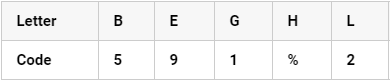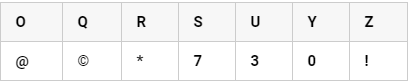Q. EOQBZ

Solution:

From rule (i), E → Z(!) and Z → E (9)

QUESTION: 22

(i) If the first two letters are vowels, the codes for first and last letters are to be interchanged.
(ii) If the first letter is a consonant, the codes for the second and third letters are to be interchanged.
(iii) If the last letter is a vowel then the second letter is to be coded as the code for the vowel.Q. GSEUZ

Solution:

From rule (ii), S → E (9), E →S (7)

QUESTION: 23

(i) If the first two letters are vowels, the codes for first and last letters are to be interchanged.
(ii) If the first letter is a consonant, the codes for the second and third letters are to be interchanged.
(iii) If the last letter is a vowel then the second letter is to be coded as the code for the vowel.Q. OESLS

Solution:

From rule (i), O →E (9), E → O (@)

QUESTION: 24

(i) If the first two letters are vowels, the codes for first and last letters are to be interchanged.
(ii) If the first letter is a consonant, the codes for the second and third letters are to be interchanged.
(iii) If the last letter is a vowel then the second letter is to be coded as the code for the vowel.Q. OHERU

Solution:

From rule (iii), H → E (9), E → H(%)

QUESTION: 25

(i) If the first two letters are vowels, the codes for first and last letters are to be interchanged.
(ii) If the first letter is a consonant, the codes for the second and third letters are to be interchanged.
(iii) If the last letter is a vowel then the second letter is to be coded as the code for the vowel.Q. EBLSO

Solution:

From rule (iii), B → L (2), L → B (5)

QUESTION: 26

Directions 26-30): Study the following arrangement and answer questions given:

A ^ D N 3 & G E 2 9 Q \$ * C S 1 ) R I 4 M @ ! Z H 5 © K L U

Q. If all the symbols are dropped from the arrangement, then which of the following is the 8th letter to the right of the 18th letter from the right end?

Solution:

The series becomes: A D N 3 G E 2 9 Q C S 1 R I 4 M Z H 5 K L U

QUESTION: 27

Study the following arrangement and answer questions given:

A ^ D N 3 & G E 2 9 Q \$ * C S 1 ) R I 4 M @ ! Z H 5 © K L U

Q. How many consonants are there in the arrangement each of which is immediately followed by a symbol?

Solution:

Q and M

QUESTION: 28

Study the following arrangement and answer questions given:

A ^ D N 3 & G E 2 9 Q \$ * C S 1 ) R I 4 M @ ! Z H 5 © K L U

Q. Complete the series: N&D E9G \$CQ ?

Solution:
QUESTION: 29

Study the following arrangement and answer questions given:

A ^ D N 3 & G E 2 9 Q \$ * C S 1 ) R I 4 M @ ! Z H 5 © K L U

Q. How many such numbers are there each of which is immediately preceded by a symbol?

Solution:

A ^ D N 3 & G E 2 9 Q \$ * C S 1 ) R I 4 M @ ! Z H 5 © K L U

there's no such number

QUESTION: 30

Study the following arrangement and answer questions given:

A ^ D N 3 & G E 2 9 Q \$ * C S 1 ) R I 4 M @ ! Z H 5 © K L U

Q. Four of the five are alike in a certain way, which does not belong to the group?

Solution:

\$ * C S 1  ------------> S1\$

E 2 9 Q \$ -------------> Q\$E

1 ) R I ------------------>  RI1

M @ ! Z H --------------> ZHM

Track your progress, build streaks, highlight & save important lessons and more!

### Similar Content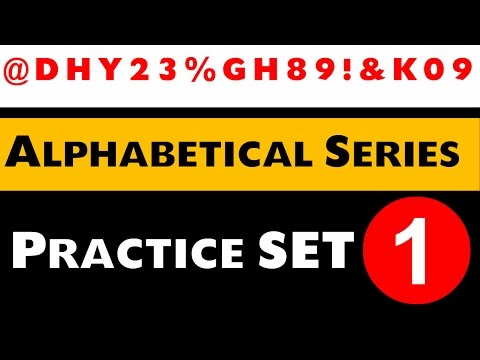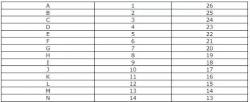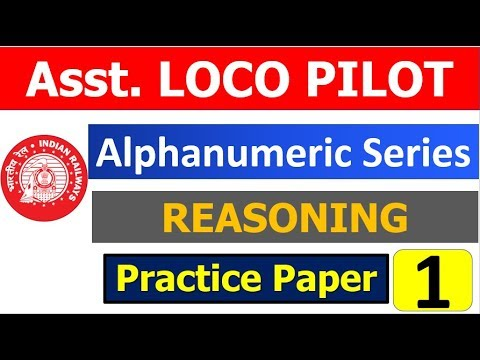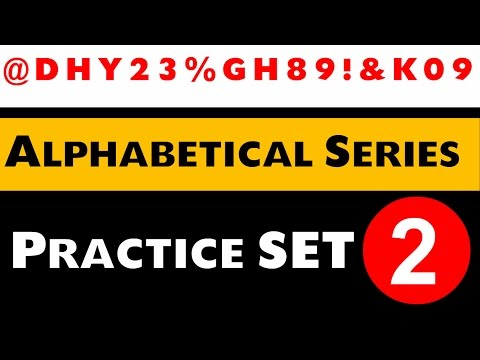### Related tests